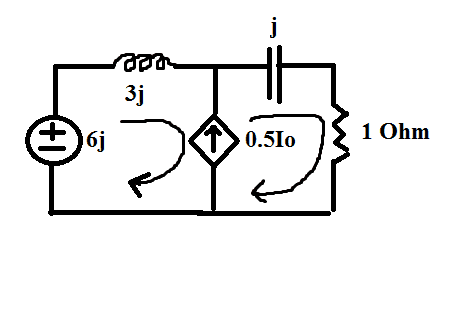# Find current using mesh analysis question?

## Homework Statement

We have the circuit in the figure.I have to find Io.## Homework Equations

Mesh current analysis.

## The Attempt at a Solution

The first mesh is i1 and the second one is i2.
We have a supermesh here.Supermesh results when two meshes have a (dependent or independent)current source in common.We exclude the current source.
We have -6j+3j*i1- j*i2+i2=0 .We also have i2=i1+ 0.5*Io
But I guess I need a third equation to solve this and to find Io,since we have three unknown currents.Whats that third equation?

gneill
Mentor
I think there's some piece of information missing from the problem statement. You do indeed need another equation or a particular voltage or current value to create one.

Can you post the problem statement in full?

This is all the possible data :(

Anyway,do you think that the above steps and equations are correct? :)

The Electrician
Gold Member
Where is Io? Is Io the current through the 1 ohm resistor (directed downward, possibly)? If so, then you have another equation, Io = I2.

Where is Io? Is Io the current through the 1 ohm resistor (directed downward, possibly)? If so, then you have another equation, Io = I2.
0.5*Io is the one in the middle,but in my exercise isnt given where is Io itself :(

gneill
Mentor
0.5*Io is the one in the middle,but in my exercise isnt given where is Io itself :(
Well, perhaps as an educated guess you could assume that Io is the current associated with the voltage source (and thus the first loop's mesh current as well). See what you get for Io in that case.

Otherwise there's not much that can be done that isn't just hand waving.